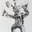Question-and-Answer Resource for the Building Energy Modeling Community
Get s tarted with the Help page

# What is the net area of thermal solar collector?In EnergyPlus, to define the net area of a PV collector (cell surface), we can specify Fraction of surface area with active solar cells.

But to define the net area of a solar thermal collector, this option doesn´t exist. So, the area of the associated solar collector surface is used in the calculations how net catchment area?

Thanks

edit retag close merge delete

Sort by » oldest newest most votedPV (and solar thermal) collectors are attached to a building surface (wall, roof, etc.) or a shading surface. The area of that surface is used to determine the size of the array along with the input for fraction of surface area.

From the Input Output Reference for PhotovoltaicPerformance:Simple "Fraction of Surface Area with Active Solar Cells":

This field is the user defined fraction for the area of surface named in the parent PV object that will have active PV cells on it. The area actually covered with solar cells will be the net area of the surface (gross area less any subsurfaces like windows) times the fraction entered here. This fraction includes the difference between PV module area and active cells within it and any losses for how closely packed modules can be arranged on surface. The value should be between 0.0 and 1.0.

So, for example, a PV array is attached to the wall of a building which contains some windows.

The gross area of the wall is 100m2, and the area of the windows is 25m2, so the net area of the wall is 75m2.

The total area of the PV modules is 50m2, and 90% of each module is active cells, so the area of active solar cells is 0.9*50=45m2.

The value for "Fraction of Surface Area with Active Solar Cells" would be 45/75 = 0.6.

more